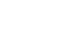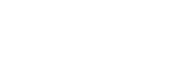Thursday, 15 December 2022 07:22

## TECHNICAL SCIENCES PAPER 2 GRADE 12 QUESTIONS - NSC PAST PAPERS AND MEMOS JUNE 2022

INSTRUCTIONS AND INFORMATION

1. This question paper consists of SIX questions. Answer ALL the questions in the ANSWER BOOK.
2. Start EACH question on a NEW page in the ANSWER BOOK.
3. You may use a non-programmable calculator.
4. You may use appropriate mathematical instruments.
5. You are advised to use the attached PERIODIC TABLE.
6. Number the answers according to the numbering system used in this question paper.
7. Show ALL formulae and substitutions in ALL calculations.
8. Round off your FINAL numerical answers to a minimum of TWO decimal places.
9. Give brief motivations, discussions etc where required.
10. Write neatly and legibly.

### QUESTIONS

QUESTION 1: MULTIPLE-CHOICE QUESTIONS
Various options are provided as possible answers to the following questions. Choose the answer and write only the letter (A–D) next to the question numbers (1.1 to 1.5) in the ANSWER BOOK, for example 1.6 D.
1.1 Which ONE of the following combinations about ketones is correct?

 STRUCTURE OF THE FUNCTIONAL GROUP NAME OF THE FUNCTIONAL GROUP AFormyl group B Carbonyl group C Carboxyl group D Hydroxyl group

(2)
1.2 Consider the structural formula of the compound below and identify the type of intermolecular forces that exist between molecules.1. Only London forces and Dispersion forces
2. Only Dispersion forces and Dipole-dipole forces
3. Only Hydrogen bonds and Dispersion forces
4. Only Dispersion forces and induced-dipole forces (2)

1.3 Consider the organic reaction below. What is represented by X and Y?
X + Y → CO2 + H2O

1. Hydrocarbon and Oxygen
2. Haloalkane and Oxygen
3. Hydrocarbon and Haloalkane
4. Oxygen and Esters (2)

1.4 Which of the following are examples of intrinsic and extrinsic semiconductors?

 Intrinsic semiconductor Extrinsic semiconductor A Silicon Germanium B Phosphorous Arsenic C Silicon Arsenic D Phosphorous Germanium

(2)
1.5 Which ONE of the following combinations of statements concerning n-type and p-type semiconductors is true?

1. n-type semiconductors have negative charge carriers whereas p-type has positive charge.
2. n-type semiconductors have negative charge carriers whereas p-type has positive charge carriers
3. n-type is formed when an extrinsic semiconductor is doped with a pentavalent impurity whereas p-type is formed when an extrinsic semiconductor is doped with a trivalent impurity.
4. n-type is formed when an intrinsic semiconductor is doped with a pentavalent impurity whereas p-type is formed when an intrinsic semiconductor is doped with a trivalent impurity.
1. (i) and (iii)
2. (ii) and (iii)
3. (i) and (iv)
4. (ii) and (iv) (2)



QUESTION 2 (Start on a new page.)
Consider the organic compounds represented by the letters A to F below and answer the questions that follow.2.1 Define the term functional group. (2)
2.2 Write down the letter(s) that represents the following:
2.2.1 A tertiary alcohol (1)
2.2.2 An unsaturated hydrocarbon (1)
2.2.3 An ester (1)
2.2.4 A Carbonyl group (1)
2.2.5 Hydrocarbons (1)
2.2.6 Chain isomers (1)
2.3 Write down the IUPAC name of:
2.3.1 C (1)
2.3.2 E (1)
2.3.3 F (1)
2.4 Write down the:
2.4.1 STRUCTURAL formula of compound D (2)
2.4.2 STRUCTURAL formula for the positional isomer of compound C such that it is a minor product (1)
2.4.3 Molecular formula of compound A (1)
2.4.4 STRUCTURAL formula for the functional group of compound B (1)


QUESTION 3 (Start on a new page.)
Students were observing the boiling points of carboxylic acids, alcohols and ketones with the same number of carbon atoms. The results are randomly recorded as follows:

 Compound Boiling point (°C) A Carboxylic Acid 222 B Alcohol 118 C Ketone 56

3.1 Which compound will have the …
3.1.1 lowest vapour pressure? (1)
3.1.2 lowest viscosity? (1)
3.2 Compounds listed above differ in boiling points owing to the strength of intermolecular forces they possess. Name the common type of intermolecular force found in all 3 groups of organic compounds in the table above. (1)
3.3 Name the type of intermolecular forces that are dominant in carboxylic acids and alcohols. (1)
3.4 Use the data in the table above and your answer in QUESTION 3.3 to explain why carboxylic acids have a higher boiling point than alcohols. (3)


QUESTION 4 (Start on a new page.)
Curves A, B and C are obtained in an investigation for a relationship between boiling points and a number of carbon atoms in straight chains of different homologous series, namely alkanes, aldehydes and alcohols.4.1 Define the underlined term in the statement above. (2)
4.2 Identify the curve (A, B or C) that will represent the following:
4.2.1 The highest vapour pressure (1)
4.2.2 The highest melting point (1)
4.2.3(1)
4.2.4 Hydrogen bonds (1)
4.3 Use the graph to draw the structural formula of a compound that will be less viscous at 573 K. (2)
4.4 The graph shows that the boiling point increases as the number of carbon atoms increases. Fully explain the trend depicted by the graph. (3)


QUESTION 5 (Start on a new page.)
The table below represents the products that were obtained in various organic reactions that were conducted. Consider the table and answer the questions that follow.

 PRODUCT: HOMOLOGOUS SERIES PRODUCT: STRUCTURAL FORMULA REACTION 1 AlcoholREACTION 2 AlkaneREACTION 3 HaloalkaneProduct for REACTION 1 was obtained when an alkene reacted with water.
Product for REACTION 2 was obtained when an alkene reacted with hydrogen.
Product for REACTION 3 was obtained when an alkane reacted with a halogen.
5.1 For REACTION 1, write down the:
5.1.1 IUPAC name of the alkene used (2)
5.1.2 Name of this reaction (1)
5.1.3 ONE reaction condition for this reaction (1)
5.1.4 Type of alcohol that this product is (1)
5.1.5 Type of product formed:
Write only MINOR PRODUCT or MAJOR PRODUCT (1)
5.2 For REACTION 2, write down the:
5.2.1 Balanced chemical equation using STRUCTURAL FORMULAE (3)
5.2.2 Name of the addition reaction represented here (1)
5.2.3 General formula for the alkenes (1)
5.3 State, with reasons, why the alkane formed is a saturated hydrocarbon. (2)
5.4 For REACTION 3, write down:
5.4.1 Name of the halogen used (1)
5.4.2 Structural formula for the positional isomer of the product formed (2)
5.4.3 IUPAC name of the positional isomer given in QUESTION 5.4.2 above (1)
5.5 Define a polymer. (2)
5.6 Give ONE use of polythene. (1)


QUESTION 6 (Start on a new page.)
A semiconductor is a material which has an electrical conductivity between that of a conductor and an insulator.
6.1 Define the following terms:
6.1.1 An intrinsic semiconductor (2)
6.1.2 Doping (2)6.2 Identify the:
6.2.1 p-type semiconductor (1)
6.2.2 n-type semiconductor (1)
6.2.3 With reference to parts marked A and B, explain the answers to QUESTION 6.2.1 and QUESTION 6.2.2. (2)
6.3 Briefly explain how the p-n junction is formed. (3)

TOTAL: 75

TABLE 3: THE PERIODIC TABLE OF ELEMENTS